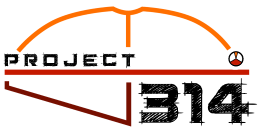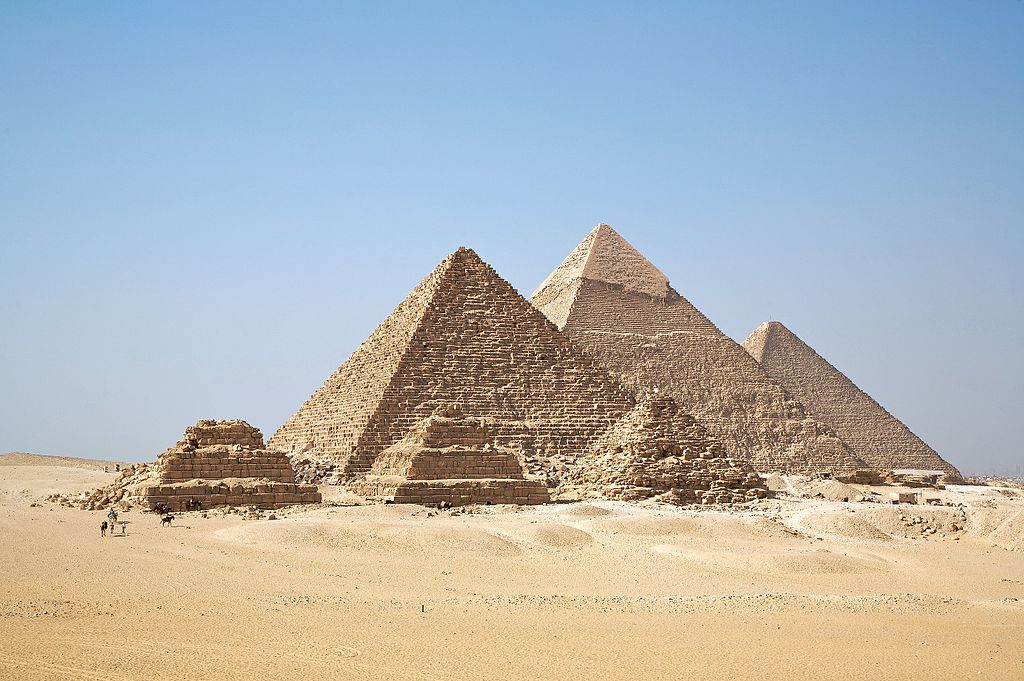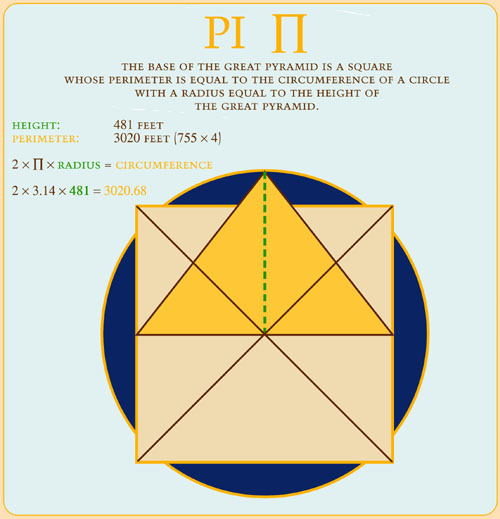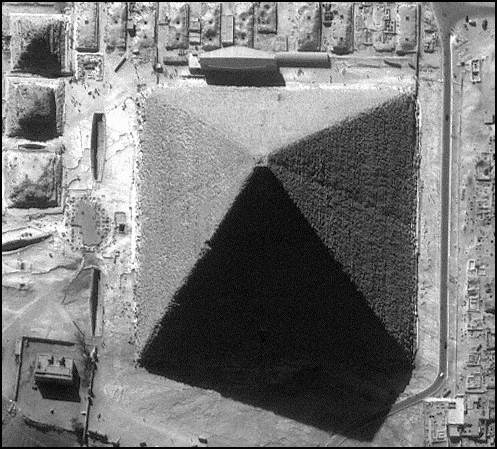# Home

## Ancient PI (π)

Ancient PI (π)
Andrew Hoy

Understanding that a circle’s circumference could be approximated by a polygon perimeter with an infinite number of sides, Viète consequentially deduced the following formula for calculating π in 1593:This formula allowed Viète to accurately approximate the constant π to nine digits, as 3.141592654.

Long before the Greek mathematician Archimedes used polygons to approximate π, it is apparent that Egyptian and Babylonian builders recorded more practical approximations of π.  For example, a Babylonian clay tablet (~1900-1600 BC) implies a value of 25 / 8, which is equal to 3.125. Also, the Rhind Papyrus of Egypt treats π as 19 / 6, which is equal to 3.1605. Nearly a thousand years later in India, Sanskrit texts recorded π as 9785 / 5568, which is equal to 3.088.  Indian mathematicians also approximated π as √10, or 3.1622.  After 100 AD, Ptolemy expressed π to four digits as 3.1416 - close enough to build almost anything in the ancient world.The Pyramids of Giza in Cairo, Egypt

Perhaps of greater relevance to the history of π is Egypt’s Great Pyramid.  Giza's wonder of the ancient world was built with a perimeter of about 1760 cubits and a height of about 280 cubits.  Some have noted that the ratio was equal to 44 / 7, which is two times the familiar 22 / 7 ratio, which is still used today to conveniently calculate π to two decimal places, or 3.14.  This Giza Pyramid geometry is summarized in the illustration below:The PI Constant as Conveyed in the Great Pyramid

While some Egyptologists try to suggest this recorded “ratio in stone” is but a matter of mere coincidence, it would be absurd to propose that people building on a scale such as Giza would not be able to arrive at 3.14 by means of empirical measurement – provided that their engineers and mathematicians were remedial. After all, the Pyramid builders aligned sides of the square pyramid base to true north within 4 minutes of arc (equal to a total of 0.067 degree), which implies that the accuracy with which they measured or surveyed was twice that of their convenient π approximation! If they had the technology to place over 2 million blocks while controlling the base dimensions of the structure to greater than 0.1% accuracy, surely they could have measured the ratio between a circle and its diameter with equal or greater precision.Ikonos satellite image of the Great Pyramid

Although several scholars have debated Israel's involvement in creating the Giza Pyramid, few would suggest that the Pyramids were not standing at the time of Israel's Exodus.  Moses was said to have been "Learned in the wisdom of the Egyptians".  While skeptics can easily dismiss the relationships between the structure's and the 22/7 ratio for π, it's hard to believe that Moses would have left Egypt at 40 years old not knowing how to calculate the circumference of a circle.

References: